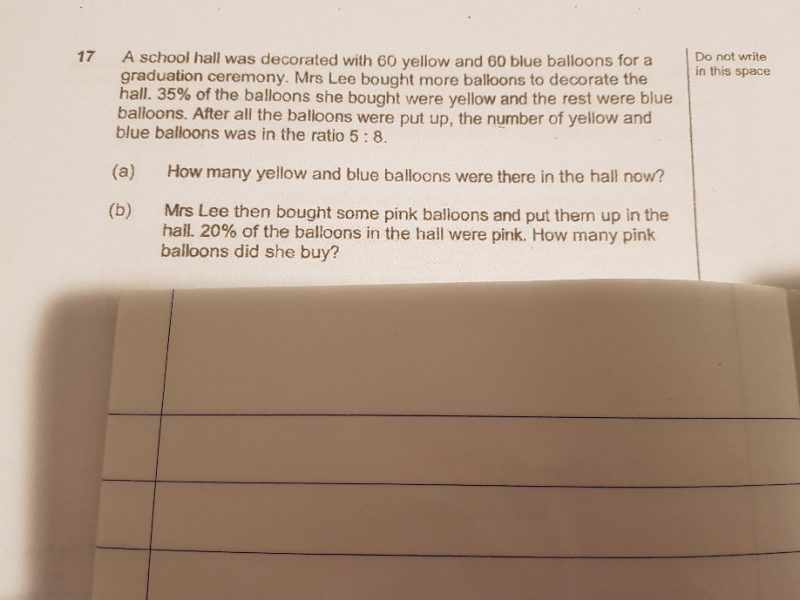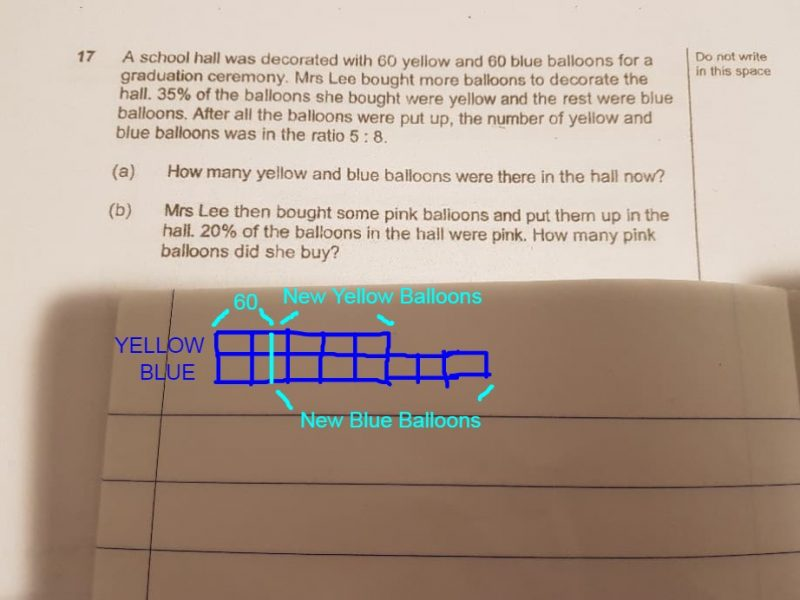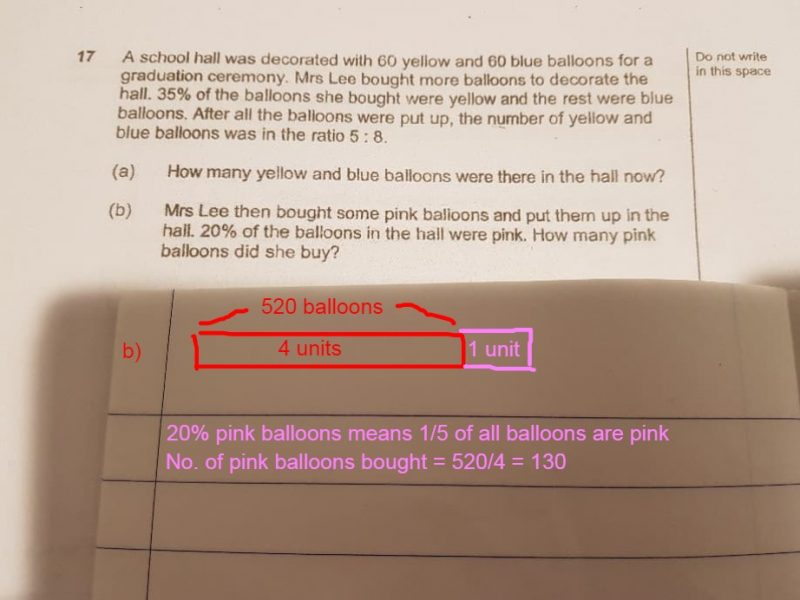# Question(a)
Method 1 :
100 – 35 = 65
65 – 35 = 30
8 – 5 = 3
30/3 = 10
60/(5 x 10 – 35) = 4 or 60/(8 x 10 – 65) = 4
60 + 60 + 100 x 4 = 520

Method 2 :
yellow balloons : 60 + 35u ——-> 5p
blue balloons : 60 + 65u ——-> 8p
30u ——-> 3p
10u ——–> 1p
60 + 35 units = 50 units
1 unit = 4
60 + 35 units + 60 + 65 units = 520

(b)
100% – 20% = 80% ——-> 520
1% ——-> 6.5
20% ——-> 130

Ans : (a) 520 yellow and blue balloons; (b) 130 pink balloons.

0 Replies 0 LikesIn response to the poster’s comments on preference not to use algebra, i have devised a different approach using the model drawing. It is slightly complicated and not sure if you can follow my train of thought.

Refer to my above model drawing.

35% of the new balloons are yellow which means

65% of the new balloons are blue

So far so good. OK. Here comes the tricky part.

We know that in the end, the blue balloons have 3 units more than the yellow balloons.

And we know that out of the additional balloons added, there is 30% more blue balloons (65%-35%)

Hence that 30% of new balloons is represented by the extra 3 units in our model drawing!

This means that every 10% of new balloons is represented by 1 unit in our drawing

Therefore the extra new yellow balloons (35%) is actually 3.5 units and the extra new blue balloons (65%) is actually 6.5 units

Since Yellow balloons has 5 units, this means that our original 60 yellow balloons is represented by 1.5 units (5 – 3.5).

Hence, 1 unit = 60/1.5 = 40

13 units = 40 x 13 = 520 balloons!

Would this approach be better?

0 Replies 0 LikesStandard disclaimer : I am not a teacher and I am not sure if P6 maths can use algebra or not. I have heard that P6 is able to use algebra but not sure the extend it is allowed in such problem sums. I approached this with algebra as it seems to be the easiest way. If it is not allowed, let me know and I see if I can do it another way.

a) Let the no. of additional balloons bought be x

Yellow balloons now -> 60+0.35x = 5 units —(1)

Blue balloons now -> 60+0.65x = 8 units — (2)

From (1), 1 unit = 12 + 0.07x —(3)

Putting (3) into (2), 60+0.65x = 8(12+0.07x)

60+0.65x = 96+0.56x

0.65x-0.56x = 96-60

0.09x = 36

x = 36/0.09 = 400

Total no. of yellow and blue balloons = 60+60+400 = 520

b) see above picture